American Journal of Modern Physics
Volume 4, Issue 3-1, May 2015, Pages: 48-52

Simulation of Radiation Spectra of Planar Channelled Electrons in Thick Silicon Crystals

Hamid Shafeghat, Alireza Abbasnia, Saeed Mohammadi

Department of Physics, Payame Noor University, Tehran, Iran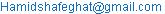(H. Shafeghat)

Hamid Shafeghat, Alireza Abbasnia, Saeed Mohammadi. Simulation of Radiation Spectra of Planar Channelled Electrons in Thick Crystals. American Journal of Modern Physics. Special Issue: Many Particle Simulations. Vol. 4, No. 3-1, 2015, pp. 48-52. doi: 10.11648/j.ajmp.s.2015040301.21

Abstract: Dechanneling processes for electrons based on the solution of Fokker-Planck equation have been studied. The dynamics of particle distribution density in depth of Crystal has been investigated in dependence on both energy and initial scattering distribution of electron beams. The influence of dechanneling process on spectral intensity of channeling radiation for electrons in crystals is investigated.

Keywords: Channeling Radiation, Fokker-Planck Equation, Dechanneling, Radiation Spectra

1. Introduction

Channeling radiation by relativistic charged particle within crossing from a monocrystal, parallel with a plane or axis of the crystal is emitted. Study on relativistic electron/positron interaction in solids under the channeling conditions is of continuous interests due to its ability to produce electromagnetic radiation well-known as channeling radiation. This type of radiation is characterized by high both monochromaticity and intensity; moreover, such sources are tunable within broad energy spectrum. Due to the principal difference in interaction potentials at channeling for electrons and positrons, the radiation sources based on positron channeling might be much powerful for positrons because of the large dechanneling length in comparison with the beams of electrons. However, till now there is an uncertainty in calculations of dechanneling processes for moderate energies .

General features of electron/positron channeling radiation at moderate energies have been recently analyzed without the details of beam dechanneling . As known at the energies higher then 100–150MeV the description of electron/positron channeling in most of the crystals (or crystallographic directions) can be done within the classical approximation (Some details of the analysis for classical and  quantum approximations at electron channeling for a specific  crystal are presented in ), the motion is characterized by the particle trajectories, which the projectiles form within the continuous potentials of crystal planes or axes at eitherplanar or axial channeling, respectively. However, the motion under the channeling regime is rather unstable due to the strong scattering processes caused by various interactions in a crystal (the perturbations by thermal atomic vibrations, electron subsystem, etc.). That is why in order to calculate the radiation intensity we have to know the beam both transverse-spatial and energy distributions in the field of continuous potential, in other words, we have to solve the diffusion equation for the beam. For light relativistic projectiles the typical diffusion equation describing the kinetics of the beam evolution down the crystal can be reduced in well-known Fokker-Planck equation [3,4].

Another solution approach to this problem based on the binary collisions method of simulations for the trajectories of channeled electrons in a crystal .

In our work we have studied the dechanneling processes for electrons in a Si crystal, based on the solution of Fokker-Planck equation [6,7]. The dynamics of particle distribution density hasbeen investigated in dependence on energy as well as initial scattering distributions of electron beams.

This method, combined with the theory developed in [8,9], was used to calculate the radiation spectra at planar channeling of relativistic electrons and positrons in thick crystals.

Monocrystals silicon for high Debye temperature as an target available and direction of relativistic electrons parallel to the planes (111), (110) and (100) is considered.Theoretical calculations and simulations to calculate the spectrum of electromagnetic radiation channel based on computer code has been done with the software Mathematica. Taking into account the transverse motion of relativistic electrons and classical equations of motion of electrons, relativistic electron trajectory calculation,Then, with the integration of the Fokker-Planck equation and the equation of classical electromagnetic radiation, the radiation spectrum of  planar relativistic electrons channeled for thick crystal is calculated.

2. Channeling Radiation Theory

Channeling motion of electrons in the crystal is estimated by averaging the planar potentional [10,11]. In Figure 1, the potential energy of interaction between the electron and the different planes (111), (110) and (100) silicon crystal is shown in the transverse direction.Transverse relativistic equation of motion of charged particles in the classical potential is given by following equation: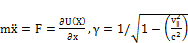(1)Figure 1. Continuous potential of electron with planes (111), (110) and (100) Silicon crystal.

Assuming the non-relativistic motion of the transverse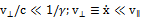. To solve the equation of motion 1, the initial conditions are: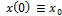And the transverse momentum of the electron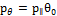that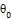The angle between the direction of the electron relative to the direction of the crystal planes (z axis).Transverse energy charged particles is given by the following equation: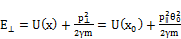(2)

3. Incident Electron Density Distribution in the Crystal in Channel

Relativistic charged particle density distribution function is obtained by the following integral:(3)

the values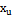,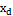withsolving the equation of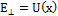for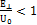and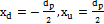for the case of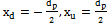calculated. Figure 2 shows initial distributionat the parallel to channeling planes incidence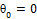for incident beam divergence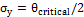. Calculations performed for electrons 1 GeV.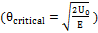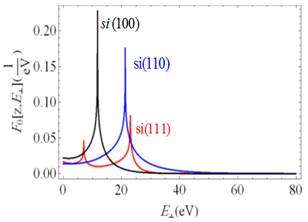Figure 2. the initial angular distribution of relativistic electrons channeled in si (111),Si(110),Si(100) at zero incident angle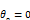.

The kinetic description of channeling phenomenon was first suggested by Lindhard using the equation of motion of diffusion type (see reference ). Successfully, Kitagava and Ohtuski have first reduced the diffusion at channeling to the solution of the Fokker-Planck equation in phase space of transverse coordinates and velocities . General expression of the Fokker-Planck equation can be written in the following form: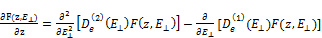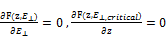(4)

in which the drift coefficient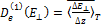is responsible for the mean transverse energy increase, while the term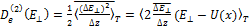is the diffusion coefficient. The coefficients are averaged by the period of motion in the channel. Both drift and diffusion coefficients have been calculated within the Kitagava-Ohtuski approximation  evaluating the integrals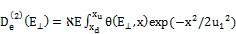(5)

and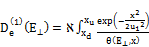(6)

where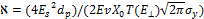is the function defined by the projectile as well as crystal parameters,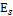is the total electron energy,is the thermal vibration amplitude, the coordinates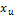,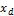are as above defined,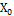is the characteristic radiation length of the crystal, the continuum potentional depth and inter planar distance are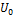,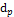respectively.

Taking into account the above mentioned conditions, the dependence of both drift and diffusion coefficients on transverse energy are shown in figure 4.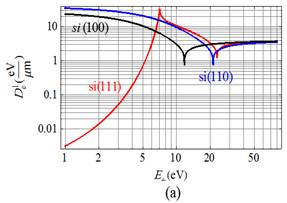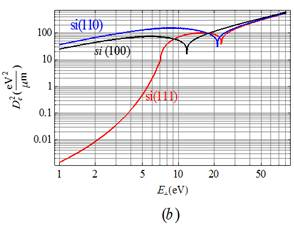Figure 3. The drift (a) and diffusion (b) coefficients of electron at planar channeling in various planes Si crystal.

It should be underlined that at transverse energy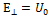, both drift and diffusion coefficients are characterized by deep minima. It takes place due to the fact that the electron density (its probability) at potentional maximum is very high. Since the nuclear density in the mid-plane between the crystallographic planes is rather low (mainly negligible), the probability of projectile scattering on nuclei becomes small. That is why kinetic coefficients reach their minima at that plane that in is contrastto the conditionfor which electron appears at a crystal plane. For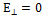the drift coefficient approches the constant value foamorphos matter, since continuous the potentional influences only very little the trajectory of and electron, while the diffusion coefficient increases linearly as function of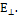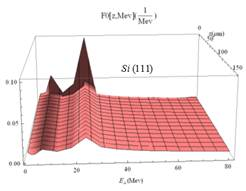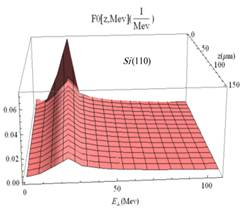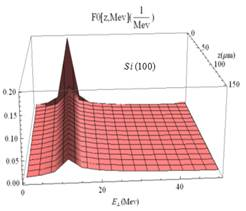Figure 4. Probability of electron density at planar channeling in Si crystal.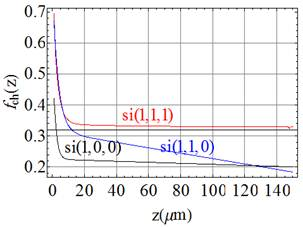Figure 5. Electrondechanneling function for the case of Si planar channeling.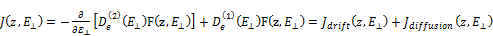(7)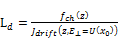(8)

z is chosen from the condition that particle flux determined by the decrease of dechanneling function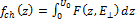in e times (figure 5):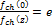This definition for dechanneling length does not depend on standard deviations in angular distribution, thus becoming the channel characteristics. For 1 GeV electrons channeling in a Si crystal the dechanneling length calculated by (8) equals to :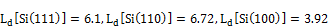4. Channeling Radiation Spectrum of Si Thick Crystal

As known the length normalized radiation intensity of relativistic charged particles at channeling in crystals is defined by the following expression [12,13]:(9)

Where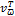is the Fourier-component of transverse particle velocity. Successively, channeling radiation taking into account the particle initial angular distribution can be evaluated as :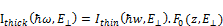(10)

However, this approach is valid only for very thin crystals. Indeed, for thick crystals we have to pay attention to the projectile dechanneling (with the crystal thickness increase the projectiles become unbound by the channel potentional due to the increase of the transverse energies), which contributes to variation of distribution function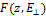. Hence, the expression for radiation intensity in thick crystals can be written as follows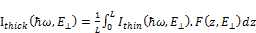(11)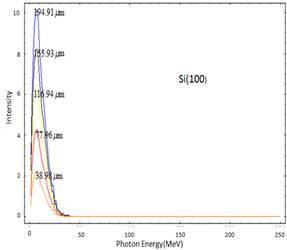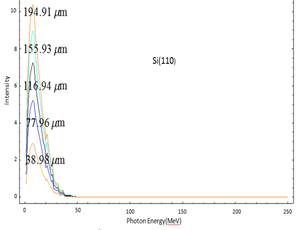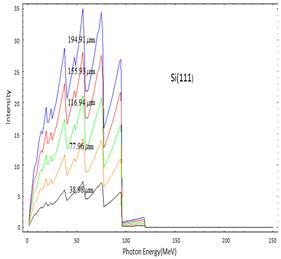Figure 6. Thickness dependence for radiation spectrum at electrons channeling in Si.

Figure 6 shows the radiation spectra of channeled electrons per unit length of the crystalline target Si.

5. Conclusion

In this work we calculation the initial angular distributions of relativistic electrons in the beam as a function of transverse energy for various angles of incidence with respect to the crystal (111),(110) and (100) planes.

For 1GeV electrons channeling in Si crystal dechanneling length evaluated from the solution of the Fokker-Planck equation. Spectral characteristics of channeling radiation of electrons of high energies in thick crystals have been first obtained taking into account the dynamics of beam scattering at channeling as well as the initial angular distribution of channeled electrons.

References

1. Carrigan R. A., Negative Particle Planar and Axial Channeling and Channeling Collimation, in the book "Charged and Neutral Particles ChannelingPhenomena- Channeling 2008", Dabagov S.B., and Palumbo L., Eds., World Scientific Publ. (2010) 129.
2. DabagovS.B., and ZhevagoN.K., NuovoCimento31(9)(2008) 491.
3. Kumakhov M.A., and Shirmer G., Atomic Collisions in Crystals, Moscow: Atomizdat, 1980 (in Russian).
4. BeloshitskyVV, KomarovFF, and Kumakhov MA, Dechanneling, flux-peaking and energy losses of fast charged particles penetrating through thick crystals, Phys. Rep. 139 (6)(1986) 293-364.
5. Kostyuk A., Korol A., Solov’yovA.and GreinerW., J. Phys. B: At. Mol. Opt. Phys. 44(2011) 075208.
6. Ohtsuki Y.-H., Charged Beam InteractionWith Solids, New York, 1983.
7. BackeH.et al. Nucl. Instr. Meth. in Phys. Res. B266 (2008) 3835.
8. BogdanovO.V., KorotchenkoK.B. and PivovarovYu.L., J. Phys. B: At. Mol. Opt. Phys. 41 (2008) 055004.
9. BabaevA.A., et al., On Crystal-AssistedProcesses by Means of 20–800 MeV e-/e+LNF Beams, PreprintLNF 22 (IR)(2008) pp. 1-42.
10. Gemmell D.S., Rev. Mod. Phys. 46(1974) 129.
11. Doyle P.A. and Turner P. S., ActaCrystallogr. A 24, 390 (1968).
12. Baier V. N., V. M. Katkov and V. M. Strakhovenko1998, Electromagnetic Processes at High Energies in Oriented Single Crystals. World Scientific, Singapore.
13. AkhiezerA.I., and Shu’lga N.F. High Energy Electrodynamics in Matter, Gordon and Breach, Luxemburg, 1996.

 Contents 1. 2. 3. 4. 5.
Article ToolsAbstractPDF(406K)